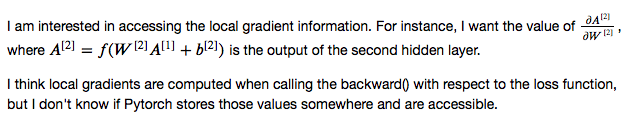Hi,

These values are not needed as is, only their product with `dL/dA` is needed. So these values are not always computed actually. It depends on the function f and sometimes the product is computed without ever creating this matrix explicitly.

These values are not needed for reverse AD which is what PyTorch uses. It instead computes values like dloss/dA2 as intermediates, which you can access using tensor hook on A2.

Thank you for your reply. Please correct me if I am wrong. Based on my understanding of backprop, local gradients are needed to compute the gradient that is used for performing the updates. For exampleAs @Yaroslav_Bulatov mentioned, the backward function computes, given a `dl/dA`, `dl/dW`.
The formula tells you that `dl/dW = dl/dA * dA/dW` but sometimes `dA/dW` has a structure such that it is not needed to actually compute it.

For the sum operation for example, if `A = W.sum()` (assuming W being 1D), then `dA/dW = 1` (a 1D vector full of ones). In such case, `dl/dW` can be computed by simply expanding `dl/dA` to the size of `W`. And `dA/dW` is never needed as a matrix to compute gradients.

But what about the cases where dA/dW is needed to be computed explicitly?

In these cases it is computed as a temporary variable and deleted before exiting the function to reduce memory consumption.

I see. Is there anyway to access that temporary variable?

You can call `.retain_grad()` on it before the backward call. That way, its .grad field will be populated.